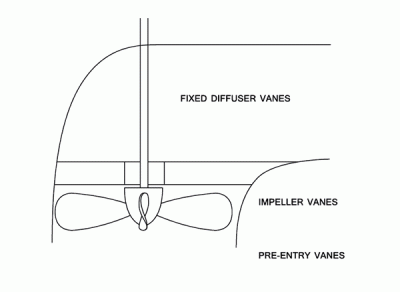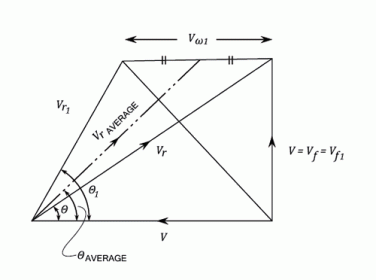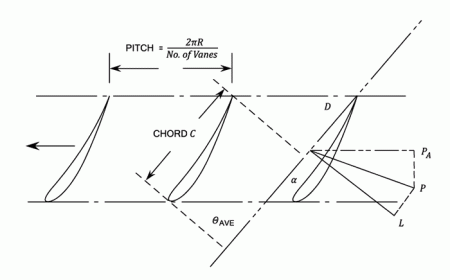•http://facebook.com/
•https://www.google.com/accounts/o8/id
•https://me.yahoo.com

# Axial Flow Pumps and Fans

Aerofoil and Euler theories applied to Axial Pumps and Fans.

## Introduction

An Axial flow pump or Fan is used to push fluid in a direction that is parallel to the shaft of the impeller. In comparison, a radial or centrifugal pump or fan would direct the fluid perpendicular to the axis of rotation. Axial pumps are sometimes termed propeller pumps owing to their similarity to the propeller of a boat. The difference, however, is that these pumps are usually shrouded in a casing to transmit fluids from one specific location to another.
Axial flow pumps are commonly used in marine applications to transfer ballast. They are also used to transfer and mix large masses of liquids in chemical treatment plants. Axial flow Fans are well adapted to air-cooled heat exchangers in industrial or airborne cooling units. They are also widely used for air circulation in large scale industrial and manufacturing units.

## Axial Flow Pumps And Fans

The figures below are for an Axial Flow Propeller fan pumping air.Leonhard Euler (15 April 1707 - 18 September 1783) was a pioneering Swiss mathematician and physicist. He made important discoveries in fields as diverse as infinitesimal calculus and graph theory.

Notes:
• The fixed diffuser vanes are used to remove the whirl component of the discharge velocity of the impeller and to convert the energy to Pressure.
• The impeller vanes may be adjustable.
• The machine may be fitted with pre-entry vanes to ensure that there is no pre-rotation and that the flow is purely Axial.
• The bottom diagram is produced by considering a Radius of the impeller and drawing it out in a flat plane.

The flow through the machine (The boss area can be neglected).

Also, (Blade area neglected).

### Euler Theory

Work done by the Vanes per lb. of water ()

Hydraulic or Manometric

Where = The Manometric head minus the Head developed by the Pump across the Flanges.

Applying Bernoulli's equation across the Vanes:
(Neglecting losses in the Vanes)

Therefore the Pressure rise across the Vanes is given by:

But,

## Aerofoil Theory Applied To Propeller Pumps

The Combined Inlet and Outlet and Inlet Triangles.The Blades are considered to be aerofoils in cascade in a fluid stream of Velocity Average in a direction to the tangent.Force exerted by the Fluid on the Vane element is the vector Sum of the lift and the force exerted on the Vane by the Fluid. The components of are in the Tangential Direction and in the Axial Direction.

Torque, also called moment or moment of force, is the tendency of a force to rotate an object about an axis, fulcrum, or pivot. Just as a force is a push or a pull, a torque can be thought of as a twist.

Consider an elemental Vane thickness at Radius .

From Aerofoil Theory Lift,

And Drag,

and are lift and drag coefficients depending upon the aerofoil section and the angle of incidence.

Resolving and in the direction of motion.

is commonly small compared with and the second terms are often neglected.

For Vanes the Total Tangential Force =
Therefore, Torque

The Horse-Power required to rotate the Vane elements =

The Efficiency = W.H.P. out put / H.P. in put

Where W = Weight of flow through the annular ring =

The Total Axial Force = If is the pressure drop across the vanes,

This equation can be solved to find .

Example:
[imperial]
##### Example - Example 1
Problem
For the Axial Flow Propeller it is required to find the torque /ft. radius using both Aerofoil and Euler Theories.

We know the radius is and the speed is
Workings
and

Theoretical Head = 114 ft. of air.

Vanes

Chord = 1.1 ft.

It is required to find the Torque per ft radius

But,

Velocity triangle:

Lift

For twelve Vanes the longitudinal Force = 12 Pt

Therefore, Torque required

Note:

Axial thrust / blade

Total axial Force for 12 Vanes =

If is the pressure rise, then

Or,

### An Alternative Approach Is:

The weight of Flow through an annular element,

Work done per lb per lb.

Therefore the work done per second per ft. radius

= Torque * Angular Velocity =

The Pressure rise across the Vanes:
Solution
The Torque required is

Last Modified: 23 Nov 11 @ 12:40     Page Rendered: 2022-03-14 08:59:51